## Antisampling for estimation: an overview

#### Neil C. Rowe

Department of Computer Science

### Abstract

We survey a new way to get quick estimates of the values of simple statistics (like count, mean, standard deviation, maximum, median, and mode frequency) on a large data set. This approach is a comprehensive attempt (apparently the first) to estimate statistics without any sampling. Our "antisampling" techniques have analogies to those of sampling, and exhibit similar estimation accuracy, but can be done much faster than sampling with large computer databases. Antisampling exploits computer science ideas from database theory and expert systems, building an auxiliary structure called a "database abstract". We make detailed comparisions to several different kinds of sampling.

Index terms for this paper: statistical computing, sampling, estimation, query processing, statisical databases, expert systems, inequalities, parametric optimization. This paper appeared in IEEE Transactions on Software Engineering, SE-11, no. 10 (October 1985), 1081-1091.  The equations were redrawn in 2008.

## Introduction

We are developing a new approach to estimation of statistics. This technique, called "antisampling", is fundamentally different from known techniques in that it does not involve sampling in any form. Rather, it is a sort of inverse of sampling.

Consider some finite data population P that we wish to study (see Figure 1). Suppose that P is large, and it is too much work to calculate many statistics on it, even with a computer. For instance, P might be one million records, too big for the main memories of most personal computers. We would have to store it on disk, requiring minutes to transfer to main memory for a calculation of a single mean. If we are in a hurry, or if we are doing exploratory data analysis and are just interested in a rough estimate of the statistic, this is too long. So we could create a sample S of P, a significantly smaller selection of items from P, and calculate statistics on S rather than on P, extrapolating the results to P.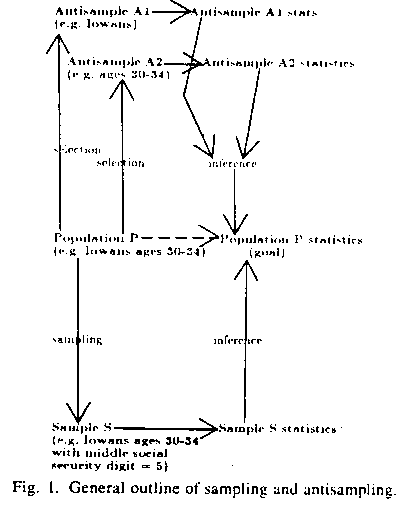But there is another way we could estimate statistics on P, by coming from the other direction. We could take some larger set known to contain P -- call it A for "antisample" -- and calculate statistics on it, then extrapolate down to P. (This terminology is suggested by the matter-antimatter dichotomy in physics; antisampling is a sort of opposite to sampling, using opposites of sampling techniques.) Downwards inference might be preferable to upwards inference from a sample, because an antisample can contain more information than a sample because it is bigger. For instance, sample S may be missing rare but important data items in population P that are in antisample A.

But there seems to be a big problem with antisampling: antisample A must be larger than population P, and it would seem more work to calculate statistics on A than P. But not necessarily. An important principle of economics is that cost can be amortized, distributed across many uses. Just as the cost of development of a package of statistical routines can be distributed over many purchasers, the work of calculating statistics on an antisample A can be charged to many uses of those statistics. We can do this if we choose an interesting antisample that people often ask questions about. Of course, we don't have to confine ourselves to one antisample; we can have a representative set of them, a "database abstract" for a particular universe of data populations or database. There are many excellent situations for amortization. For instance, U.S. Census aggregate statistics on population and income are used by many different researchers for many different purposes; and laws require periodic publication of this information anyway.

Two caveats regarding these techniques are necessary, however. First, a form of the "closed world assumption" important in database research  is necessary: we can only make inferences about the population from which the antisamples are drawn, and not any larger population. This means that if the populations themselves are samples (a "hybrid" approach of antisampling and sampling) we cannot make inferences about the larger populations from which those samples are drawn, which makes the hybrid approach not very useful. Second, like sampling, antisampling (almost always) gives estimates. Since antisampling and sampling are rather different methods, sometimes the estimation accuracy of antisampling is better than sampling, sometimes not. However, antisampling is much faster than sampling with large computer databases, and this is its main justification. Generally, antisampling is a good idea when one of three conditions hold: (1) users are doing exploratory data analysis, the initial stages of statistical study; (2) users are statistically naive; or (3) data is predominantly kept on secondary (magnetic or optical disk) or tertiary (magnetic tape) storage.

## The analogy of antisampling to sampling

### Areas of correspondence of antisampling and sampling

Analogs of nearly all the same techniques can be used with antisampling as with sampling. For instance if the sum statistic on a sample S is T, then the extrapolation rule for the sum statistic inferred on the population P is T times the ratio of the size of P to the size of S. Similarly, if a sum statistic on an antisample is T the extrapolated estimate of the statistic on P is T times the ratio of the size of P to the size of A. Sampling and antisampling can also both combine estimates based on multiple samples and multiple antisamples using stratification and other methods. With antisampling, a study population is typically specified as the intersection of several antisample "parent" sets.

Antisampling is in fact a more natural direction for making inferences, since the inference rules (equivalently, "estimation methods") used with sampling are often derived from assuming a class of distributions representing the data population and reasoning what characteristics of the sample would be, then inverting this and reasoning backwards. So antisampling rules are derived, then inverted to get sampling rules. Thus we expect less uncertainty associated with antisampling than sampling. Note also another reason: sampling requires assumptions of the form of the distribution from which the sample is drawn, while antisampling does not use such information. But there is a concomitant disadvantage of antisampling: the population about which inferences are drawn will not usually be random with respect to the antisamples. We can assume it is random to get "reasonable-guess" estimates of statistics, but this will get us into trouble when different attributes of the data are strongly correlated and the query mentions the correlated attributes. Another approach is to store many correlation (linear or nonlinear) statistics about an antisample so that the randomness of a population within an antisample may be estimated. These complexities have a partial compensation in bounding capability, a special property of antisampling not shared by sampling, discussed in the next section.

One important aspect of antisampling deserves emphasis, however. Unlike sampling, antisampling is knowledge-intensive: it requires construction of a special auxiliary structure, the database abstract. This makes antisampling systems like the expert systems of artificial intelligence , requiring for construction careful cooperation of experts in the domain of the data. This is because the choice of just what data to put in the database abstract is important. One could just parameterize the distribution of each attribute, then parameterize the two-dimensional distribution for each pair of attributes, and so on, as  does, but this exhaustive approach fails to take advantage of many redundancies between the various distributions involved. After all, there are an infinite number of possible statistics, subsets, and attributes (included derived ones) on a finite database, and even with strong complexity limits on queries the combinatorial possibilities can be immense. Correlations between attributes can be quantified as statistics too, by regression coefficients. Expertise with the data is thus required to advise what statistics best summarize it. This must be traded off with the frequency that users ask particular sorts of queries (perhaps weighted by utilities of query answers to them). Both normative (e.g. mean) and extremum (e.g. maximum) statistics are desirable for the abstract, to characterize both the common and the uncommon in the data, since users will want to ask about both. Important sets of related items formed by partitioning the values of each attribute should be the basis for statistics (what we in  call "first-order sets", and what  calls "betas").

But the database abstract does have a certain degree of flexibility. If a user insists on a different partitioning of data values on some attribute than the partitioning of the abstract, we can find a superset of abstract partitions that includes the user's and use sampling theory. For instance, if the user asks about people age 27-33 when we only have partitions for ages 25-29 and 30-34, we calculate exact statistics on the union set for ages 25-34, estimate the number of people 27-33, and estimate their characteristics treating them as a sample of the set on the larger range. (If we know correlational information between age and an attribute of interest, the sample need not be random.)

### Absolute bounds and production systems

Antisampling supports a different kind of inference virtually impossible with sampling: reasoning about absolute bounds on statistics. Suppose we know the maximum and minimum of some attribute of an antisample A. Then since P must be contained entirely within A, any maximum, minimum, mean, median, or mode of P is bounded above and below by the maximum and minimum on A. But you can't do this the other way around: given the maximum and minimum of a sample S, you have no idea what the largest possible value or smallest possible value on the population P is for the maximum, minimum, mean, median, or mode on P. With particular assumptions about P and S you can put confidence limits on statistics of P -- say if you assume that S is a random sample drawn from P, and that P doesn't contain any extreme outliers, the mean of S will tend to be close to the mean of P, with a certain standard deviation. But assumptions like these, common in statistics, are messy and uncomfortable for computer scientists. There is a qualitative difference between being 95% sure and being completely sure. If one can obtain a tight absolute bound, it should be preferable to a confidence interval.

But a serious objection may be raised to absolute bounds as opposed to confidence intervals: they can sometimes be very weak because they must account for a few highly extreme but possible cases. There are four answers to this. First, many uses of statistics do not require high degrees of accuracy. If one is doing exploratory data analysis, the statistic may just be used to get an an idea of the order of the magnitude of some phenomenon in the database, and absolute bounds within an order of magnitude are quite satisfactory  Also, there are situations where statistics are used for comparison, and the only question is whether the statistic is greater than or less than a value, as in choosing the best way to process a database retrieval from one of several equivalent methods based on estimated sizes of the sets involved .

Second, absolute bounds often are easier to calculate than reasonable estimates. The usual need for distributional assumptions means many more parameters in estimating than bounding. A good demonstration is in section 4.3. Using the same mathematical approach for both, estimates lead to nonlinear equations with exponentials and no closed-form solution, whereas bounds lead to polynomials that can be handled with standard symbolic optimization methods to obtain closed-form expressions. The easier computability of bounds has long been recognized in computer science, as in the theory of algorithms where worst-case analysis using the O notation is more common than the complexities of probabilistic modelling required for average-case analysis.

Third, absolute bounds can be made tigher with associated assumptions of reasonable ranges for other unspecified statistics. For example, Chebyshev's inequality says that no more than a fractionitems can lie more than D from the mean of a distribution. But if the distribution has a single mode close to the mean, the Camp-Meidell inequality (Camp, 1922) gives results about twice as good.

The fourth reason that possibly weak absolute bounds on the value of statistics can still be useful is an important insight in the field of artificial intelligence: many small pieces of weak information can combine to give strong information. And with absolute bounds on quantities the combining is easy; just take the minimum of the upper bounds, and the maximum of the lower bounds, to get cumulative upper and lower bounds; no distributional or independence assumptions are required. Often very different kinds of reasoning can lead to different bounds on the same quantity, and it is unnatural and inelegant to combine all these different methods into a single formula. The next section gives some examples.

Expressing reasoning methods as a number of small, isolated pieces of information is the idea behind the artificial-intelligence concept of a "production system" , a programming architecture that has been applied to many interesting problems. It is the opposite extreme to the notion of a computer as a sequential processor, as for instance in an optimization program that uses a single global measure to guide search for a solution to a complicated problem. In a production system there is no such global metric, only pieces of separate knowledge about the problem called "production rules", all competing to apply themselves to a problem. Production systems are good at modeling complex situations where there are many special cases but no good theory for accomplishing things. Thus reasoning about absolute bounds given statistics on antisamples seems a natural application for production systems. It has some similarities to symbolic algebraic manipulation, which often uses this sort of architecture . We can use a number of more sophisticated techniques developed in artificial intelligence to avoid redundant computation in a production system, as for instance relaxation methods or "constraint propagation" . We can also write estimation methods as rules, and combine both estimates and bounds into a comprehensive system.

## A short demonstration

To show a little of what this approach can accomplish, we show some behavior for a partial Interlisp implementation, as of February 1983. (We have done work since then, but have not yet integrated it into a complete system.) The database contained information on about 600 merchant ships on a day in 1977. The database abstract includes simple statistics on all first-order (single-word-name) sets, including statistics on each ship nationality, ship type, and major geographical region. No correlations between attributes are exploited. "Guess" is the estimate; "guess-error" the standard deviation associated with that estimate; "upper-limit" and "lower-limit" are the absolute bounds on the answer. The "actual answer" is found by going afterwards to the actual database and computing the exact value of the statistic. The system does not understand English -- we have just paraphrased our formal query language to make it easier to read. For more details and demonstrations see .

` `
`How many French ships of type ALI are there?`
`(GUESS: 6.2 GUESS-ERROR: 2.3 UPPER-LIMIT: 10 LOWER-LIMIT: 3)`
`(ACTUAL ANSWER IS 7)`
` `

What's the mean longitude of a Liberian tanker of type END?

(GUESS: 45.4 GUESS-ERROR: 34.5 UPPER-LIMIT: 168 LOWER-LIMIT: 3)

How many type ALI tankers are either French or Italian?

(GUESS: 12.6 GUESS-ERROR: 3.3 UPPER-LIMIT: 63 LOWER-LIMIT: 3)

` `
`What's the frequency of the most common tanker class`
`among the French, Italian, American, and British?`
`(GUESS: 18.5 GUESS-ERROR: 2.2 UPPER-LIMIT: 25 LOWER-LIMIT: 15)`
`(ACTUAL ANSWER IS 18)`
` `

What's the mean longitude for Liberian ships of type ALI

not in the Mediterranean?

(GUESS: 49.6 GUESS-ERROR: 42.4 UPPER-LIMIT: 176 LOWER-LIMIT: 6)

` `
`What's the mean distance of ALI-type ships from 30N5W?`
`(GUESS: 51.0 GUESS-ERROR: 12.3 UPPER-LIMIT: 57.1 LOWER-LIMIT: 6.0)`
`(ACTUAL ANSWER IS 42.34673)`
` `

What's the most common registration-nationality region

for type ALI ships currently in the Mediterranean?

(GUESS: 46.6 GUESS-ERROR: 9.3 UPPER-LIMIT: 78 LOWER-LIMIT: 26)

## Three examples

In this short paper it is impossible to describe the varied categories of inference rules on antisample statistics that we have studied.  and  provide overviews, and the latter provides additional details and many examples. But for illustration we present three important categories.

### Bounding the size of set intersections

Set intersections (or equivalently, conjunctions of restrictions on a query set) are very common in user queries to databases. Efficient processing requires good methods for estimating their counts or sizes in advance.

If we know the sizes of the sets being intersected, then an upper bound on the size of the intersection is the minimum of the set sizes. A lower bound is the sum of the set sizes minus the product of the size of the database and one minus the number of sets being intersected, or zero if this is negative.

We can do better if we have more statistics on the antisamples. If we know the mode frequencies and number of distinct values on some attribute, then an upper bound is the product of the minimum mode frequency over all sets with the minimum number of distinct values of a set over all sets. Sometimes this bound will be better than the upper bound in the last paragraph, and sometimes not. We can see that if the two minima occur for the same set, the bound will be more than the size of that set, since the product of a mode frequency and number of distinct values for a single set must be more than the size of a set. On the other hand, consider two sets of sizes 1000 and 2000, with mode frequencies on some attribute 100 and 500 respectively, and with numbers of distinct values 50 and 5 respectively. Then the simple bound of the last paragraph is 1000, but the frequency-information bound is min(100,500) * min(50,5) = 500 which is better (smaller). So both approaches are needed.

We can generalize this method to cases where we know more detailed information of the frequency distributions of the sets. We just superimpose the frequency distributions and take the minimum of the superimposed frequencies for each value. See Figure 2.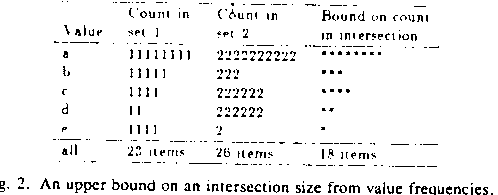If instead (or in addition to) frequency information we have maxima and minima on some attribute, we may be able to derive bounds by another method. An upper bound on the maximum of a set intersection is the smallest of the maxima on each set, and a lower bound is the largest of the minima on each set. Hence an upper bound on the size of an intersection is the number of items in the entire database having values between that cumulative maximum and minimum. If the maxima are all identical and the minima are all identical, then the cumulative maximum and minima are the same as on any of the sets being intersected, so the simple (set-size) bound will always be better. But the maximum-minimum bound can be an excellent one whenever two or more of the sets being intersected have very different ranges, as when we are intersecting two sets with ranges 100 to 500 and 450 to 750 respectively, and the cumulative range is 450 to 500, and there are few items in the database with those particular values -- we can then impose an upper bound on the intersection size. See Figure 3.We can also use sums (or equivalently, means) on attributes. Suppose: (1) we wish to estimate the size of the intersection of only two sets; (2) one set is a partition of the database for the values of some numeric attribute; (3) we know all values this attribute can have; and (4) know the size and mean of both sets. Then we can write two linear Diophantine (integer-solution) equations with the number of items having each possible value of the attribute being the unknowns, and solve for a finite set of possibilities. We can then take the minima of the pairs of the maximum possible values for each values, and sum to get an upper bound on the size of the intersection. Diophantine equations tend to support powerful inferences, since the integer-solution constraint is a very strong one. There turn out to be many related phenomena that can give additional constraints on the variables, making inferences even better. See  for details.

Several other kinds of reasoning can bound the size of set intersections as discussed in .

### Bounding the means of monotonically transformed values

Suppose we know the means and standard deviations of some antisamples. Suppose we are interested in the logarithms of the original data values. (Sometimes different transformations on the same data values are all useful, or sometimes we may not be sure when we create the antisamples what the best transformation is, or sometimes different ranges of the data values require different transformations for best analysis.) And suppose we are interested in knowing the mean of the transformed data values.  examines this problem in detail; we summarize it here.

A variety of classical techniques has been applied to this problem. For instance, you can approximate the logarithm curve by a three-term Taylor-series approximation at the mean, giving as an estimate of the mean of the logarithms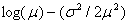.  But it is hard to obtain confidence intervals on this result to quantify its degree of uncertainty, though several methods have been tried . This estimate is always biased, and sometimes is an impossibility (when it gives a value unachievable with any possible distribution consistent with the original mean and standard deviation).

Rule-based inferences about bounds provide an appealing alternative. Several simple methods bound the mean of the logarithms, no one the best for all situations. We can try them all, separately for upper and lower bounds, and combine results. .BR

1. Linear approximation bounds. We can draw lines that lie entirely above or entirely below the the function we are approximating on an interval. For many curves, the best upper bound line is found by taking the tangent at the mean, and the best lower bound line is found by drawing a secant across the curve from the smallest data value to the largest data value. See Figure 4.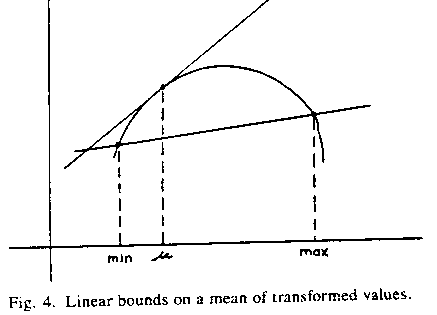A. Taylor-series. That is, bounds curves from first three terms of a Taylor-series about some point on the function.

B. Chebyshev-Lagrange. That is, a quadratic LaGrange interpolating polynomial passing through the three points of the function that are optimal for Chebyshev approximation.

C. Special-purpose. For particular functions (e.g. reciprocal and cube), particularly tight bounds because of peculiarities of the mathematics of those functions.

D. Pseudo-order-statistic. Taylor-series approximations improved by Chebyshev's inequality and related inequalities.

3. Order statistics. If we know medians or quantiles we can break up the approximation problem into subintervals corresponding to each quantile range, and solve a subproblem on each.

4. Optimization. We can iteratively converge to optimal bounds for a class of bounding curves, by expressing the class parametrically and optimizing on the parameters, with objective function the statistic being bounded. This tends to be computationally expensive and not advisable when estimation speed is important.

As an example, suppose we know the minimum of the set of data is 10, the maximum is 20, the mean is 15, and the standard deviation is 1. Then the linear bounds on the mean of the logarithm are 2.650 and 2.708; the Taylor-series bounds found by taking the Taylor-series at the mean are 2.689 and 2.716; the LaGrange-Chebyshev's bounds are 2.701 and 2.709; the Pseudo-Order-Statistics bounds are 2.700 and 2.711; and the best quadratic bounds found by optimization are 2.703 and 2.708. For another example, suppose the minimum is 1, and maximum is 200, the mean is 190, and the standard deviation is 20. Then the linear bounds are 5.032 and 5.247; the Taylor-series bounds are 1.484 and 5.242; the LaGrange- Chebyshev's bounds are 2.948 and 5.499; the pseudo-order-statistics bounds are 3.363 and 5.242; and the bounds found by quadratic optimization are 5.032 and 5.242. These bounds are surprisingly tight, and should be adequate for many applications.

There is a more direct optimization method for this problem, involving treating the optimization variables as the values of a distribution satisfying certain constraints and moving the variables around until an optimum is achieved. We have experimented with such optimization, but it is considerably less well-behaved than the parametric one mentioned earlier. It is tricky to get to converge properly, even in simple situations. This optimization also suffers from serious sensitivity to errors in calculation. And since we can only use a small number of variables compared to the sizes of many interesting populations, the number converged to by the optimization process will be only a lower bound on an upper bound, or an upper bound on a lower bound, and these things are considerably less helpful to us than the upper bounds on upper bounds and lower bounds on lower bounds obtained with the rule-based inferences discussed above. This is a fundamental weakness of these "direct" optimization methods, and an important justification for our approach.

### Optimal rules relating statistics on the same distribution

Another category of rules relates statistics on the same attribute of the same set (as when one estimates or bounds the mean given the median). Many of these situations may be seen as instances of the so-called "isoperimetric problem" of the calculus of variations (, ch. 4), for which there is a general solution method. The mathematics becomes complicated even for some rather simple problems, but the rules generated are mathematically guaranteed to be the best possible, an important advantage.

The idea is to find a probability distribution that has an extreme value for either some statistic or the entropy of the distribution, and then find the extreme value. Let the probability distribution we are trying to determine be y = f(x). Suppose we have some integral we wish to maximize or minimize: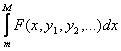Suppose we have prior constraints on the problem as known statistics expressable as integrals:where j goes from 1 to k, the total number of known statistics. As before, the limits m and M represent the minimum and maximum on the distribution, or at worst lower and upper bounds respectively on these quantities; these are necessary for this method to work, and they must be the same for all integrals.

As examples of statistics expressible as integrals:

` `
`mean:`
` `
`variance: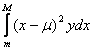`

` `
`root mean square error: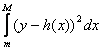`

` `
`median: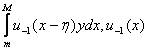the unit step function`

It was proved by Lagrange (, p. 51) that a necessary condition for an extremum (either maximum or minimum) of the F integral is

` `
`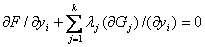`

If the F is y*log(y), this method gives a necessary condition for the maximum-entropy distribution. Several researchers have used this to obtain maximum-entropy estimates of unknown moment statistics from knowledge of other moment statistics, in both the unidimensional and multidimensional cases (, Appendix). For the unidimensional case, the form of the maximum entropy distribution given moments up through the rth is

`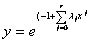`

The remaining problem is to determine the lambdas (Lagrange multipliers), which can be tricky. A number of arguments in  justify the term "optimal" for these estimates.

F can also be a statistic itself. For instance if F is the kth moment when we know values for all moments up through the (k-1)th, the necessary condition for a solution becomes:

`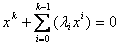`

This is a kth-order polynomial, with a maximum of k solutions. Hence the probability distribution that gives the extrema of the kth moment is a k-point discrete probability distribution. It can be found by a symbolic optimization process with 2k unknowns (k values of x, and k associated probabilities) with k equality constraints in the form of the known k-1 moments plus the knowledge that the probabilities must sum to 1.

## Detailed comparision: antisampling vs. sampling

We now evaluate the relative merits of sampling and antisampling. We assume data populations stored in computers (a condition that is becoming increasingly common with routine administrative data). The main advantage of antisampling is clearly speed of calculation, with both methods giving roughly the same estimation accuracy.

Most of our arguments concern the relative efficiency of various kinds of sampling vs. antisampling. But first some general points:

(1) Sometimes the data is already aggregated. Much of the published U.S. Census data is -- it provides privacy protection for an individual's data values. So we must use antisampling methods in some form if we want to estimate statistics not in the original tabulation -- we have no other choice.

(2) Sampling is poor at estimating extremum statistics like maximum and mode frequency. Extremum statistics have important applications in identifying exceptional or problematic behavior. Antisampling handles such statistics well, in part because it can use extremum statistics of the entire database as bounds.

(3) Updates to the database can create difficulties for samples, since the information about what records the samples were drawn from will usually be thrown away. For antisampling with many statistics including counts and sums, however, the original data is not needed: the antisample statistics can be updated themselves without additional information.

### Experiments

We have conducted a number of experiments comparing accuracy of antisampling with simple random sampling, using randomly generated queries on two rather different databases, as reported in chapter 6 of . It is hard to summarize the hundred or so complicated experiments we performed. But generally speaking, when the same amount of space was allocated to antisampling and simple random sampling (that is, the size of the database abstract was the same as the size of the sample) we found estimation performance (the closeness of estimates to actual values) roughly similar in most cases, and better for antisampling the rest of the time. This is hardly suprising considering the duality of sampling and antisampling methods. Both exploit low-redundancy encodings of typically high-redundancy database, so we expect their information content and suitability for estimation to be similar. An occasional better performance of antisampling seems due to bounds.

We have also conducted more specific experiments with the set intersection bounds of section 4.1 , and the transformation mean bounds of section 4.2 . All three sets of experiments did not measure computation time because the test databases were too small, but we expect that this will be the major advantage of antisampling with large data sets, as we now discuss.

### Simple random sampling and paging

We are currently seeing two important tendencies in statistical analysis of data sets on computers : a shift from large multi-user computers to small personal computers, and a continued increase in the size of data sets analyzed as success has been achieved with smaller data sets. Both make it increasingly impossible for analysis, or even calculation of a mean, to be carried out in main memory of a computer, and secondary storage issues are increasingly important. This is significant because secondary storage like magnetic disks and optical disks, and tertiary storage like magnetic tape, is organized differently from main memory: it is broken up into "pages" or "blocks" that must be handled as a unit. This is not likely to change very soon, as it follows from the physical limitations of secondary and tertiary storage. So since transfer of pages from a secondary storage device to a central processor takes orders of magnitude (typically, a factor of 1000) more than the operations of that processor or transfers within main memory, paging cost is the only cost of significance in statistical analysis of large data sets.

This has important implications for sampling methods because they are much less efficient when data is kept in secondary storage than main memory. Consider simple random sampling without replacement. We can use Yao's standard formula  to estimate the number of pages that need to be retrieved to obtain k sample items, assuming items are randomly distributed across pages, in just the same way the formula is used for any set randomly distributed across pages. Let p be the number of items on each database page, and let n be the number of items in the entire database. Then the formula is:We have tabulated approximations to this function for some example values in Figure 5, using the formula of  which is much easier to evaluate while having a maximum error for this range of values (reading off the tables in that paper) of less than 0.1%. We assumed a million-record database. We used two values for page size: p=100, which suggests a record-oriented database with perhaps ten attributes per record, and p=1000, which suggests the transposed file organization common with statistical databases. As may be observed, the number of pages retrieved, essentially the access cost for data in secondary storage, is close to the size of the sample for small samples. It only becomes significantly less when the sample size approaches the total number of pages in the database, in which case the number of pages retrieved approaches the number of database pages, a situation in which sampling is useless. So simple random sampling is going to be approximately p times less page-efficient than a full retrieval of the entire database, which means 100 or 1000 times worse for our example values of p. The obvious question is thus: why not just calculate the statistic on the database and not bother with the inexact answer provided by sampling?But antisampling does not share this great paging inefficiency. Assuming all statistics of attributes of each antisample are stored together on the same physical page -- a requirement usually easy to fulfill since there are not many useful statistics one can give for a set as a whole -- only one page need be retrieved for each antisample used. Usually this is a small number. If we choose a good group of antisamples, we can specify many populations users ask about in terms of set operations -- intersection, union, and complement -- on the sets covered by the antisamples, or at worst proper subsets of those antisamples. For instance, if we want to know the mean of the American tankers in the Mediterranean, and we have antisamples for every major nationality, major ship type, and region of the oceans, we need only retrieve three pages: the page with statistics about American ships, the page with statistics about tankers, and the page with statistics of ships in the Mediterranean. In general, if it is possible to express a population P in terms of K antisamples, we need only retrieve K pages, independent of the size of P, the sizes of the antisamples, or the size of the database. So as the database increases, the relative advantage of antisampling to sampling increases.

### Further difficulties with simple random sampling

Three additional problems complicate the use of simple random sampling relative to antisampling. First, it is usually desirable that sampling be without replacement, and additional algorithms and data structures are needed to ensure this .

Second, we have so far we have ignored the effort to locate members of a data population on pages in the first place, which can add further paging costs. If we have no index or hash table, we simply must examine each page in turn, throwing out the ones that have no population members, and this increases the number of pages fetches. For small populations, this means a high wastage probability that can easily be greater than the size of the sample. So it seems desirable to access a population through an index or hash table whenever possible. But an index may be too big to reside in main memory, and have paging costs itself. Usually database indexes link together items having the same value for one particular attribute at a time, so if a data population P of interest is specified by a number of restrictions on a number of different attributes, many pages of index may have to be retrieved followed by a lengthy intersection operation of the set of all pointers to data items. Hashing can more easily avoid extra paging, but usually allows access on only one attribute, which means it is not much of a performance boost in most database systems.

Third, many statistical databases are not stored by record or "case" but in the "transposed" form (, section 4-1-4), where only values for one attribute for different items (or some small subset of the total set of attributes) are stored on a page. This is an efficient form of storage for calculation of counts and means on a single attribute because there are more values of that attribute per page. But it usually doesn't help sampling because the only sampling ratios that justify sampling, based on our above arguments, tend to be very small, much less than the reciprocal of the number of items per page. Increasing the number of items per page by transposition can only increase this by a small factor in most cases (at best the ratio of the size of a full record to the size of an attribute), which will often still result in only one item being fetched per page. Transposition also slows all queries involving several attributes not on the same page.

### Rejoinder 1: randomized databases

These disadvantages of simple random sampling are clear and it may be wondered whether some other kind of sampling could be more competitive with antisampling. After all, an enormous amount of research has gone into devising a wealth of sampling techniques. Unfortunately, other techniques seem to have other disadvantages.

Consider for instance "randomizing" the database - that is, putting data items onto pages in a random way. To get a random sample then one could take all the items on just a few pages, and not just a few items on many pages, and save in paging . (Note that randomizing an index to the data would do not good -- the actual data item fetches are what are expensive.) But this is harder than it sounds. A policy has to be followed long before the data are used, requiring much shuffling on additions, deletions or changes to the data, for correlations of data with time are common and must be guarded against. Also, randomization only pays off when queries put no restrictions on the data population. With tight restrictions, you will have to look at many pages anyway just to find enough data items to satisfy them, even if the database has been randomized.

But there is an even more serious objection to randomization of a database: it degrades the performance of the database for anything other than sampling, since no longer can you put related items together on the same page. This is serious because most large databases are multi-purpose to justify their expense, used for instance for routine clerical operations for data entry as well as statistical study. Even for a database used only by statisticians, randomization hurts performance for calculation of statistics on complete non-sample sets.

### Rejoinder 2: a separate database for sampling

Since sampling is so antithetical to usual operations of the database, it might be moved to a separate machine, or copied to new structures inside the same machine, and done away from the original data. Extracting the sample may be much work considering our arguments of the previous sections, but once done the cost can be amortized over multiple queries, provided multiple queries can be asked, which depends on the needs of the querier and the usefulness of the data.

But extracted samples are less flexible than extracted antisamples. If after studying some sample S we decide we need to look at a superpopulation of the original population P, or sibling population of P (a population with a common superpopulation with P), we must go back to the database and resample all over again to obtain a new sample to analyze -- we cannot use any part of our old sample in the superpopulation sample because clearly it is biased in relation to the new sample. On the other hand, if we chose an original data population P that was too large (even though S is a comfortable size) and decide we want to focus in on some subpopulation of it, merely censoring out items in S that do not belong to the subpopulation may give too small a set for statistical analysis, particularly if the new subpopulation is quite a bit smaller than the old. In other words, sampling is "brittle": the results of one sampling are difficult to extend to a related sampling.

But antisampling extends gracefully to related populations. Adding another restriction to restrictions defining a set is usually straightforward, and can never worsen bounds obtained without the restriction -- and the the parts of the previous analysis can be reused. Similarly, removing a restriction introduces no new problems since analysis of the new population was a subproblem studied in reasoning about the original population. This accommodation of related user queries by antisampling is because much statistical analysis focuses on meaningful sets, not random sets, and antisamples are sets.

### Rejoinder 3: stratified and multistage sampling

Given the disadvantages of randomizing the physical placement of items in a database, we might take the opposite course and place items on pages in systematic ways. To sample we could use the same techniques people use in sampling a real world where data items cluster in different ways [1,5]. For instance, if pages represent time periods, we could do a two-stage sampling where we choose first random periods represented by random pages, and then random items within those pages. Or in a population census database, if pages represent particular pairs of geographical locations and occupation, we could do a stratified sampling within carefully chosen geographical-occupational combinations.

But there are many problems with using such sampling paradigms:

1. They are not for amateurs. Much knowledge about the nature of the data is necessary to use them properly -- perhaps only by an expert statistician should, and even then models of the data must be reconfirmed carefully. This can mean extensive prior statistical study of related data, as in the first example above where we must be sure that the times chosen are truly random, or in the second example where the geographical-occupational combinations must be valid strata.

2. It is hard to quantify our certainty that proper conditions pertain, and it is therefore difficult to put standard deviations on the estimates obtained by these samples.

3. If the data change with time their correlational properties may also change. Changes can cause problems with pages overflowing or becoming too sparse, requiring awkward immediate rearrangements of the partitioning scheme.

4. We can only cluster (group semantically related items together) along one dimension at a time. For instance, if we group bills by date, we cannot simultaneously group them by geographical location. This is awkward because a good partitioning for stratified sampling to study one attribute is not necessarily a good partitioning for another attribute -- database stratification is permanent unlike survey design stratification. And grouping records by "hybrid" criteria based on different dimensions of the data is hard to analyze.

5. Complex sampling paradigms are limited to certain statistics. For instance, stratified sampling only works well with "additive" statistics such as count and sum that can be totalled over disjoint partitions to get a cumulative statistic, as well as certain ratio statistics.

6. Complex sampling paradigms may require additional page access. In order to find the right pages for stratified sampling or multistaged clustered sampling, one needs "metadata"  describing the data and its storage, and the size of this often requires it be in secondary storage. Metadata is useful for many other purposes such as access method selection and integrity maintenance, so there can be a good deal of it for a database. It also makes sense to keep it with indexes to the data, if any, and these may have to be kept in secondary storage anyway.

7. The comparision of stratified and multistage sampling to simple antisampling is unfair because there are more sophisticated kinds of antisampling that correspond to the more sophisticated kinds of sampling. For instance, "stratified antisampling" can be done where we partition a population into disjoint pieces as with stratified sampling, but then use antisampling techniques to make the estimate on each piece, combining the results as with stratified sampling. See Figure 6. If the pieces are chosen to minimize intrapiece variation, the result can be better than that for simple antisampling. Sometimes stratified sampling will be better than stratified antisampling, and sometimes vice versa, in the same way that sampling compares with antisampling depending on how well the nature of the database is captured in the database abstract.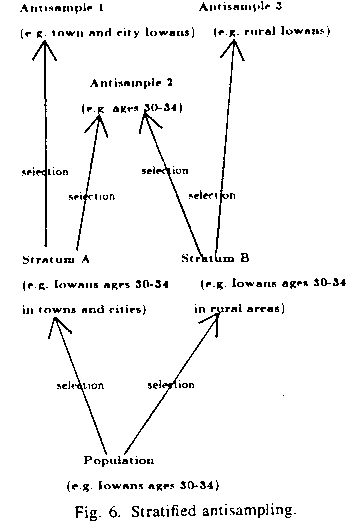In summary, difficult administrative issues in both statistical analysis and database design are raised by these more complicated sampling designs, and people with the necessary expertise are scarce. It may be on this reason alone will not be used, because if one cannot be sure one is getting a random sample then all the conclusions one draws from that sample are suspect.

### Rejoinder 4: special-purpose hardware

So far we have assumed conventional hardware. If not, statistical calculations can be faster, but this does not necessarily make sampling any more attractive.

For example, we can use logic-per-track secondary storage devices (surveyed in ). We can put hardware in the head(s) of a disk so that it calculates the sum of all items on a track within one revolution of the track, or calculates the sum of selected items by marking the items on one revolution and summing them on the next. The idea can work for any moment statistic, or maximum and minimum, but other order and frequency statistics are not additive in this sense and do not appear to be computable this way. So we can speed calculation of some statistics, perhaps additionally with parallelism in read operations on different disks or tracks, if we can afford a special-purpose "moment-calculating" disk, which is likely to be expensive because of the limited demand. But such a device would speed calculation of the exact statistic on the data too, hastening construction of a database abstract. Construction might be very efficient because it can be done by a single pass through all tracks of the disks in a disk-based database, an intensive utilization of each track.

Similarly, multiple disks or multi-head disks could enable faster statistical calculations since operations could be done on several devices in parallel. But this doesn't make the paging problem go away -- it just makes paging faster. And it makes database abstract construction simultaneously faster.

But there is one hardware development that will improve the position of sampling relative to antisampling: larger main memories that can hold larger databases. Antisampling can still be performed in this situation (and can be thought of as a form of caching), but the paging advantage disappears. Other advantages do not disappear, however. And database sizes are increasing too.

## Conclusions

We are developing a new technique for estimating statistics, primarily statistics on databases. This "antisampling" is not just another sampling method, but something fundamentally different, and subject to quite different advantages and disadvantages than sampling. We have presented some of the advantages and disadvantages of antisampling in detail. One disadvantage not yet mentioned is the mass of details that remain to be worked out. Considering the enormous amount of effort over a hundred years in the perfection of sampling techniques, much more work is clearly needed to make antisampling techniques a routine part of a statistical analysis arsenal.

## References

Author's address: Department of Computer Science, code 52, Naval Postgraduate School, Monterey CA 93943.

The work reported herein was partially supported by the Foundation Research Program of the Naval Postgraduate School with funds provided by the Chief of Naval Research. It was also partially supported by the Knowledge Base Management Systems Project at Stanford University under contract #N00039-82-G-0250 from the Defense Advanced Research Projects Agency of the United States Department of Defense. The views and conclusions contained in this document are those of the author and should not be interpreted as representative of the official policies of DARPA, the Navy, or the U.S. Government. Helpful comments were provided by Bill Gale, Richard Hamming, Frank Olken, Daryl Pregibon, Gio Wiederhold, and referees.

 W.G. Cochran, Sampling Techniques, third edition, Wiley, New York, 1977.

 R. Davis and J. King, "An overview of production systems", in E. Elcock and D. Michie (eds.), Machine Intelligence 8, Wiley, New York, l976, 300-334.

 E.C. Freuder, "Synthesizing constraint expressions", Communications of the ACM, 21, ll, 958-966, November 1978.

 J. Grant and J. Minker, "On optimizing the evalutaion of a set of expressions, International Journal of Computer and Information Science, ll, 179-191, 1982.

 M. Hansen, W. Hurwitz and W. Madow, Sample Survey Methods and Theory: Volume I, Wiley, New York, 1957.

 F. Roth-Hayes, D.A. Waterman and D.B. Lenat (eds.), Building Expert Systems, Addison-Wesley, Reading, Mass., 1983.

 M.H. Hoyle, "Transformations -- an introduction and a bibliography", International Statiistical Review, 41, 2, 1973, 203-223.

 E. Lefons, A. Silvestri, and F. Tangorra, "An analytic approach to statistical databases", Proceedings of the Ninth Internatioal Conference on Very Large Databases, Florence, Italy, October 1983, 260-274.

 J. McCarthy, "Metadata management for large statistical databases", Proceedings of the International Conference on Very Large Databases, Mexico City, Mexico, September 1982, 234-243.

 J.P. Morgenstein, "Computer based management information systems embodying answer accuracy as a user parameter, Ph.D. thesis, University of California at Berkeley, December 1980.

 R.A. Reiter, "On closed world databases", In H. Gallaire and J. Minker (eds.), Logic and Databases, Plenum, New York, 1978, 55-76.

 N.C. Rowe, "Top-down statistical estimation on a database", Proceeding of the ACM-SIGMOD Annual Meeting, San Jose, California, May 1983, 135-145.

 N.C. Rowe, "Rule-based statistical calculations on a database abstract", Report STAN-CS-83-975, Stanford University Computer Science Department, June 1983 (Ph.D. thesis). Chapter 6 also appeared in the Proceedings of the Second International Workshop on Statistical Database Management, Los Altos, California, 235-244, September 1983 (Lawrence Berkeley Laboratory publication LBL-16321).

 N.C. Rowe, "Diophantine inferences on a statistical database", Information Processing Letters, 18, 1984, 25-31.

 N.C. Rowe, "Absolute bounds on the mean and standard deviation of transformed data for constant-derivative transformations", Technical report NPS52-84-006, Department of Computer Science, U.S. Naval Postgraduate School, April 1984.

 N.C. Rowe, "Absolute bounds on set intersection sizes from distribution information", Technical report, Department of Computer Science, U.S. Naval Postgraduate School, June 1985.

 J.E. Shore and R.W. Johnson, "Properties of cross-entropy maximization", IEEE Transactions in Information Theory, It-27, 4, July 1981, 472-482.

 S.W. Song, "A survey and taxonomy of database machines", Database Engineering, 4, 2, December 1981, 3-13.

 R.C. Tobey, "Symbolic mathematical computation -- introduction and overview", Proceedings of Second Symposium on Symbolic and Algebraic Manipulation, March 1971, 1-15.

 P.F. Velleman, "Data analysis on personal computers: thoughts on the coming revolution in statistical computing", Proceedings of the Statistical Computing Section, American Statistical Association, Washington, D.C., August 1982, 36-39.

 R. Weinstock, Calculus of Variations, McGraw-Hill, New York, 1952.

 K.Y. Whang, G. Wiederhold and D. Sagalowicz, "Estimating block accesses in database organizations: a closed noniterative formula", Communications of the ACM, 26, ll, 940-944, November 1983.

 G. Wiederhold, Database Design, 2nd edition, McGraw-Hill, New York, 1983.

 C.K. Wong and M.C. Easton, "An efficient method for weighted sampling without replacement", SIAM Journal of Computing, 9, 1, 111-113, February 1980.

 S.B. Yao, "Approximating block accesses in database organizations", Communications of the ACM, 20, 4, 260-261, April 1977.

Captions for figures

Figure 1: General outline of sampling and antisampling

Figure 2: An upper bound on an intersection size from value frequencies

Figure 3: Range restriction bounds on an intersection

Figure 4: Linear bounds on a mean of transformed values

Figure 5: Number of pages needed to get k random sample items from a million-record database, using approximation of 

Figure 6: Stratified antisampling